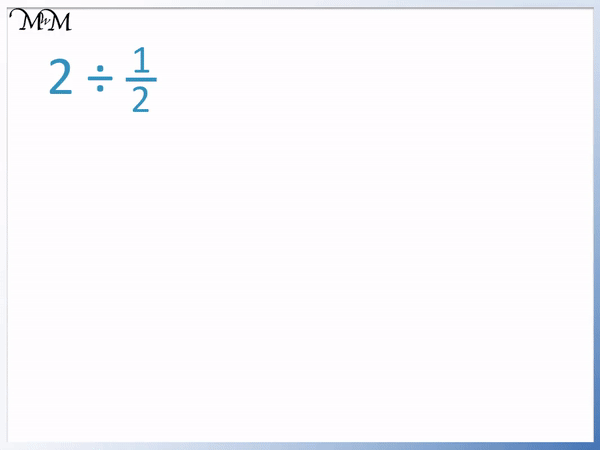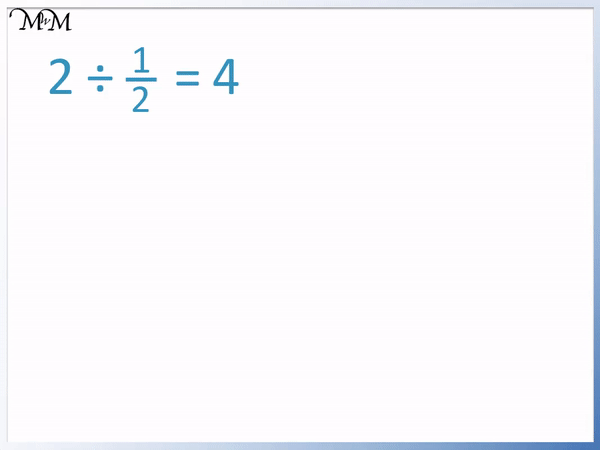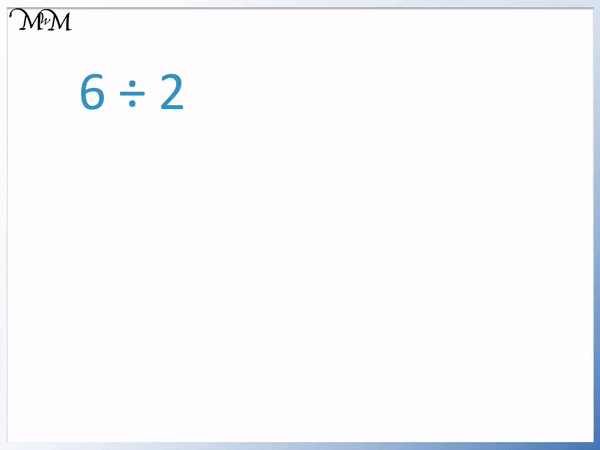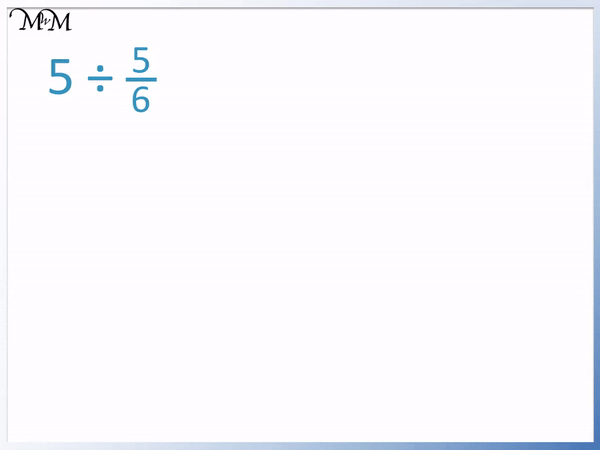# Dividing Whole Numbers by Fractions

Dividing Whole Numbers by Fractions• 2 ÷   1 / 2   is the same as asking, “How many halves go into 2?”
• We can count and see that 4 halves make up 2 wholes.
• 2 ÷   1 / 2   = 4 because   1 / 2   goes into two 4 times.• We keep the whole number the same.
• We turn the division into a multiplication.
• We flip the fraction we are dividing by.
• 2 ÷   1 / 2   becomes 2 ×   2 / 1  .
• To work this out, first multiply the whole number by the numerator on the top of the fraction.
• 2 × 2 = 4.
• Then divide this by the denominator on the bottom of the fraction.
• 4 ÷ 1 = 4.
• 2 ÷   1 / 2   = 4.
• This means that one half goes into two 4 times or that 4 halves make 2.

Turn the division into a multiplication and flip the fraction you are dividing by.

Multiply the whole number by the top of the fraction and then divide by the bottom of the fraction.• We keep the whole number 6 the same.
• We flip the fraction we are dividing by.
• 6 ÷   2 / 3   becomes 6 ×   3 / 2  .
• We multiply the 6 by the numerator on the top.
• 6 × 3 = 18.
• We divide 18 by the denominator on the bottom of the fraction.
• 18 ÷ 2 = 9.
• 6 ÷   2 / 3   = 9.# Dividing Whole Numbers by Fractions

## What does it Mean to Divide Whole Number by a Fraction?

Dividing a whole number by a fraction tells us how many of these fractions add together to make the whole number.

When teaching division by fractions, it is always best to start with a visual model.

We can first look at the division of whole numbers by whole numbers to remember what the division tells us.

Here we have 6 ÷ 2.

This division means, “How many twos go into 6?”We can see that 2 goes into six 3 times. Therefore 6 ÷ 2 = 3.

We can think of division by fractions in a similar way.

Here we have 2 ÷  1 / 2  shown with a model.

This division means, “How many halves go into 2?”It can help to teach division of fractions using a visual model.

Each whole is made up of two halves. We can count and see that 4 halves make 2 wholes.

We can see that the 2 in the whole number can be multiplied by the 2 in the fraction to give us our answer of 4.

Whilst visual models are useful for first introducing division by fractions, it is useful to have a quicker, written method.

Division is the opposite of multiplication and so, we can turn the division into a multiplication provided that we flip the fraction.

We use the three steps of:

• Keep
• Multiply
• Flip

to divide whole numbers by fractions.We keep 2 as 2.

We turn the division into a multiplication.

We flip the fraction  1 / 2  to become  2 / 1 .

We can multiply the whole number by the number on top of the fraction and divide by the number on the bottom of the fraction to work this out.

2 × 2 = 4

and 4 ÷ 1 = 4

2 ÷  1 / 2  = 4.

This means that 4 of the fraction  1 / 2  make up the whole number of 2.

Here is another model of dividing whole numbers by fractions.

We have 6 ÷  2 / 3 .

This question is asking, “How many of the fractions  2 / 3  make 6 wholes?”

The fraction of two thirds is 2 out of 3. We can see this fraction shown in the model below. every 2 blocks shaded is another two thirds.We can see that there are 9 separate lots of two thirds that can fit inside 6 wholes.

6 ÷  2 / 3  = 9 because  2 / 3  goes into six 9 times.

We can see that we can use our 3 steps of

• Keep
• Multiply
• Flip

to divide this whole number by a fraction and get the same answer.We rewrite 6 ÷  2 / 3  as 6 ×  3 / 2 .

We multiply 6 by the 3 on top and then divide it by the 2 on the bottom to get our answer.

6 × 3 = 18 and then 18 ÷ 2 = 9.

This is the same answer we got by drawing our visual model but it is more of a methodical process that does not require a drawing.

## How to Divide a Whole Number by a Fraction

To divide a whole number by a fraction:

1. Keep the whole number the same.
3. Flip the fraction you are dividing by.
4. Multiply the whole number by the number on the top of this flipped fraction.
5. Divide this result by the number on the bottom of the flipped fraction.

We will use these steps to work out the following example. We have the division of the whole number 5 by the fraction   5 / 6  .

The first step is to keep the whole number 5 as 5.

The second step is to turn the division sign, ÷, into a multiplication sign, ×.

The third step is to flip the fraction   5 / 6   to   6 / 5  .5 ÷   5 / 6   can be written as 5 ×   6 / 5  .

To work this out, we use use steps 4 and 5.

The fourth step is to multiply the whole number, 5, by the numerator on the top of the flipped fraction, which is 6.

5 × 6 = 30

The fifth step is to divide this result by the denominator on the bottom of the flipped fraction, which is 5.

30 ÷ 5 = 6

And so, 5 ÷   5 / 6   = 6.

Here is another example of dividing whole numbers by fractions.

We have 8 ÷   1 / 4  .

We keep the 8 as 8 and turn the division into a multiplication.

We flip the fraction 5 / 6   to become 6 / 5  .8 ÷   1 / 4   can be written as 8 ×   4 / 1  .

We multiply the 4 by 8 to get 32.

Then we divide 32 by 1, which is still 32.

8 ÷   1 / 4   = 32.

This means that 32 quarters make 8 wholes in total.Now try our lesson on Translating Shapes where we learn what it means to translate a shape.error: Content is protected !!